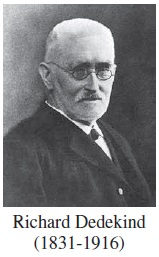Home | | Maths 11th std | Basic Algebra: Introduction

# Basic Algebra: Introduction

Indian mathematicians like Brahmagupta (598-670) and Bhaskaracharya (1114-1185) had made contributions to the understanding of the real numbers system and algebra.

Introduction

Algebra is a branch of mathematics in which one expresses relations among quantities by using symbols to represent these quantities. The symbols are called the variables. In this class we shall allow the variables to represent real numbers only. One can carry out manipulations and computations using variables just as one does with numbers. That is, one may substitute real numbers for the variables in the expression and the resulting value will also be a real number. Once a quantity or a mathematical statement is expressed in terms of variables, it is possible to substitute specific numerical values for those variables. This makes algebra a very powerful tool. For this reason the subject of algebra has very wide application, not only within mathematics, but also in other disciplines and in real life. The notion of real numbers is fundamental to the whole of mathematics. The real number system was well understood only in the nineteenth century. The need for extending the rational numbers arose quite early in the history of mathematics. Pythagoreans knew that √2 was not a rational number. Certain constructions involving irrational numbers can be found in Shulbha Sutras, which date back to around 800 BCE. Aryabhata (476-550) had found approximations to π.

Indian mathematicians like Brahmagupta (598-670) and Bhaskaracharya (1114-1185) had made contributions to the understanding of the real numbers system and algebra. In his work Brahmagupta had solved the general quadratic equation for both positive and negative roots.

Bhaskaracharya solved quadratic equations with more than one unknown and found negative and irrational solutions. The most important real number zero was the contribution by Indians.

Rene Descartes (1596-1650) introduced the term “real” to describe roots of a polynomial equation distinguishing them from imaginary ones. A rigorous construction of real number system was due to Richard Dedekind (1831-1916).Tags : Mathematics , 11th Mathematics : UNIT 2 : Basic Algebra
Study Material, Lecturing Notes, Assignment, Reference, Wiki description explanation, brief detail
11th Mathematics : UNIT 2 : Basic Algebra : Basic Algebra: Introduction | Mathematics